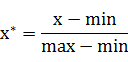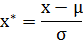# min-max标准化（Min-Max Normalization）min-max标准化python代码如下：

import numpy as np

arr = np.asarray([0, 10, 50, 80, 100])
for x in arr:
x = float(x - np.min(arr))/(np.max(arr)- np.min(arr))
print x

# output
# 0.0
# 0.1
# 0.5
# 0.8
# 1.0
• 1
• 2
• 3
• 4
• 5
• 6
• 7
• 8
• 9
• 10
• 11
• 12
• 13

# Z-score标准化方法import numpy as np

arr = np.asarray([0, 10, 50, 80, 100])
for x in arr:
x = float(x - arr.mean())/arr.std()
print x

# output
# -1.24101045599
# -0.982466610991
# 0.0517087689995
# 0.827340303992
# 1.34442799399

# 前言

====================================

# Python

## STEP1、导入依赖包

import numpy as np
import matplotlib.pyplot as plt
import pandas as pd
• 1
• 2
• 3

==>
numpy系统是python的一种开源的数值计算扩展。

==>
matplotlib.pyplot是用来做数据的展示。也就是数据的可视化。

==>
pandas该工具是为了解决数据分析任务而创建的。pandas 纳入了大量库和一些标准的数据模型，提供了高效地操作大型数据集所需的工具。
pandas提供了大量能使我们快速便捷地处理数据的函数和方法。它是使python成为强大而高效的数据分析环境的重要因素之一。

========http://blog.csdn.net/wiki_su/article/details/78404808

## STEP2、读取数据

Spyder中，设置好文件路径。千万不要忘了。#import dataset
dataset = pd.read_csv('Data.csv')
• 1
• 2#取出所有的行，（去掉最后一列 purchased）
X = dataset.iloc[:,:-1].values

Y = dataset.iloc[:,3].values
• 1
• 2
• 3
• 4
• 5## STEP3、遗失数据的处理

1、通过数据中的最大值和最小值，我们把中间值赋值给缺失的数据；
2、通过所有数据的平均值来赋值给缺失的数据；
3、删除有空缺数据项；

============

#预处理impoter
from sklearn.preprocessing import Imputer

#做遗失的部分的处理 用NaN的方式填补上来
#axis：传0或者。0代表处理列 ，1代表处理行。
#strategy的值有mean，median，most_frequent ，分别代表：平均数 中间值 最常出现的数值
imputer = Imputer(missing_values = 'NaN',strategy = 'mean', axis = 0)

#1-3行缺失的部分补上
imputer = imputer.fit(X[:,1:3])

#返回处理好的数据
X[:,1:3] = imputer.transform(X [:,1:3])
• 1
• 2
• 3
• 4
• 5
• 6
• 7
• 8
• 9
• 10
• 11
• 12
• 13

from sklearn.preprocessing import Imputer这行代码运行过。## STEP4、数据明确化

1.能够处理非连续型数值特征。
2.在一定程度上也扩充了特征。

#数据明确
#提高运行的速度和准确性
#把数据明确化，变成数字
#OneHotEncoder 热编码
from sklearn.preprocessing import LabelEncoder,OneHotEncoder

#把城市数据进行数字明确化
labelencoder_X = LabelEncoder()
X[:,0] = labelencoder_X.fit_transform(X[:,0])

#热编码，拿出来做数组的编排
onehotencoder = OneHotEncoder()
X = onehotencoder.fit_transform(X).toarray()

#把结果数据进行城市化
labelencoder_Y = LabelEncoder()
Y = labelencoder_Y.fit_transform(Y)
• 1
• 2
• 3
• 4
• 5
• 6
• 7
• 8
• 9
• 10
• 11
• 12
• 13
• 14
• 15
• 16
• 17## STEP5、数据分割

#数据分割
from sklearn.cross_validation import train_test_split

#0 取一次就好了，不要每次都取两组随机的数字
X_train,X_test,Y_train,Y_test = train_test_split(X,Y,test_size = 0.2,random_state = 0)
• 1
• 2
• 3
• 4
• 5

X_train、Y_train
X_test 、Y_test# STEP6、数据缩放

from sklearn.preprocessing import StandardScaler

#缩放
sc_X = StandardScaler()
X_train = sc_X.fit_transform(X_train)
X_test = sc_X.fit_transform(X_test)
Y_train = sc_X.fit_transform(Y_train)
Y_test = sc_X.fit_transform(Y_test)
• 1
• 2
• 3
• 4
• 5
• 6
• 7
• 8## END 完整代码

#数据预处理 data preprocessing

import numpy as np
import matplotlib.pyplot as plt
import pandas as pd

#import dataset

#Matrix of feature
X = dataset.iloc[:,:-1].values
Y = dataset.iloc[:,3].values

#预处理impoter
from sklearn.preprocessing import Imputer

imputer = Imputer(missing_values = 'NaN',strategy = 'mean', axis = 0)
imputer = imputer.fit(X[:,1:3])
#返回处理好的数据
X[:,1:3] = imputer.transform(X [:,1:3])

#数据明确
from sklearn.preprocessing import LabelEncoder,OneHotEncoder

labelencoder_X = LabelEncoder()
X[:,0] = labelencoder_X.fit_transform(X[:,0])

onehotencoder = OneHotEncoder()
X = onehotencoder.fit_transform(X).toarray()

labelencoder_Y = LabelEncoder()
Y = labelencoder_Y.fit_transform(Y)

#数据分割
from sklearn.cross_validation import train_test_split

#0 取一次就好了，不要每次都取两组随机的数字
X_train,X_test,Y_train,Y_test = train_test_split(X,Y,test_size = 0.2,random_state = 0)

#缩放X
from sklearn.preprocessing import StandardScaler

sc_X = StandardScaler()
X_train = sc_X.fit_transform(X_train)
X_test = sc_X.fit_transform(X_test)
Y_train = sc_X.fit_transform(Y_train)
Y_test = sc_X.fit_transform(Y_test)

©️2019 CSDN 皮肤主题: 编程工作室 设计师: CSDN官方博客